/
Blogs

# QuadTree in Unity

Implementation of a QuadTree data structure in unity. Here we discuss the simplest way to create data structure and implement it as well.

First of all, I wanted to share why I created such a tree. My objective was very simple : to partition the space as per the mesh density based off vertex data. Once I know which are denser areas in a given area, I can have more control over it. Refer to the following image which shows how a particular scene can be divided into quad based structure: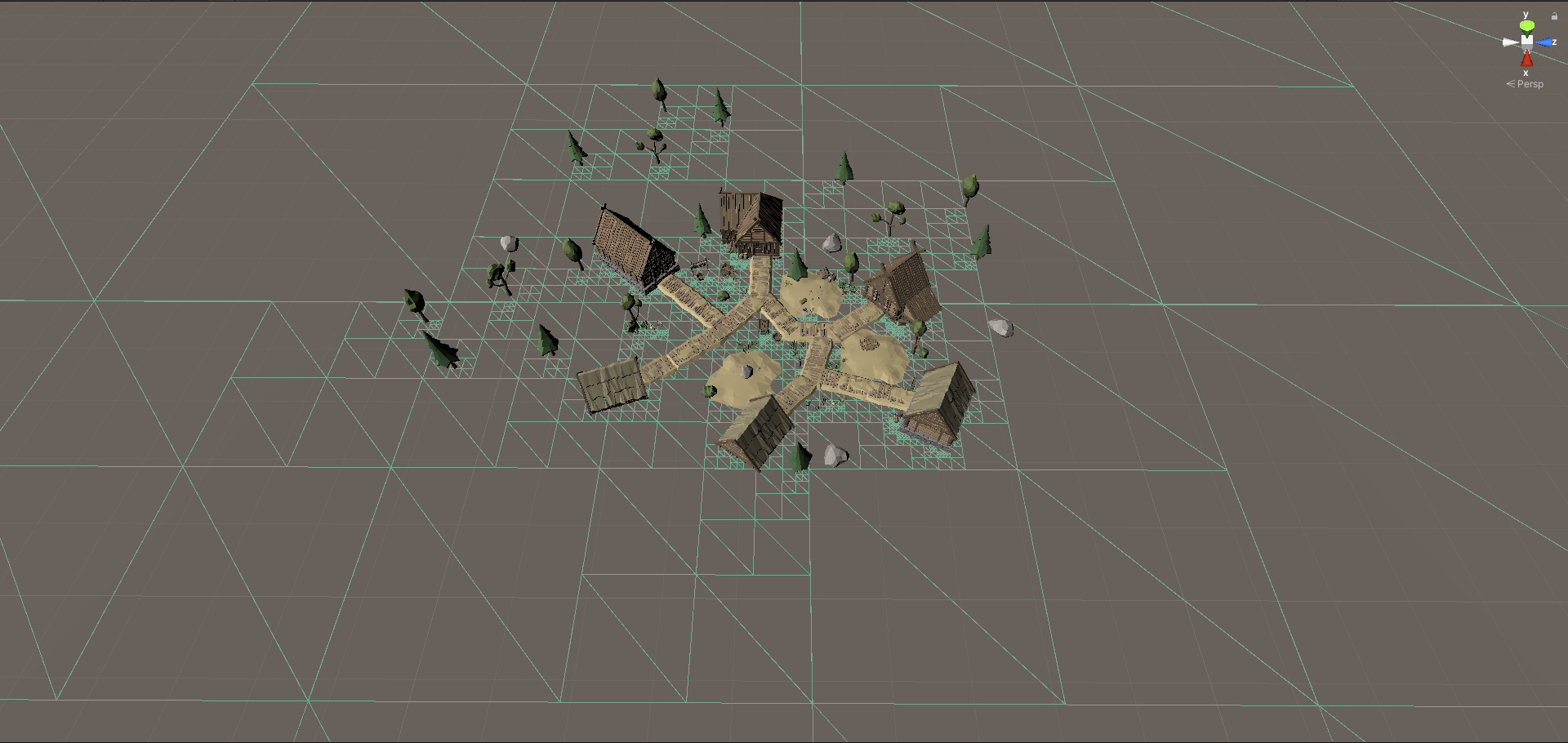# Quadtree Structure and Class

Quadtree : A quadtree is a tree data structure in which each internal node has exactly four children.

Now we have a starting point where we know that, if we start off with the root node, it would contain exactly 4 children nodes. For each child node, there will be 4 child nodes too.

Infinite nodes? If each node were to have 4 child nodes, then yes, the node structure will be infinite. We will look at how to control this in further sections.

The pseudo-structure of a quadtree can be as simple as what you can see in the image. This is all you need to create a tree structure with branches and leaves.

I have used the class-name as “Quad” to make it easier to understand. Lets see the various properties: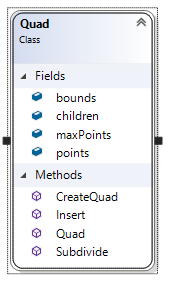Bounds : The area that the quad consists of. Each point will be checked if it lies in the bounds of the quad or not.

Children : If the number of data in a specific quad overflows, it would lead to the quad being subdivided into smaller sections. As explained earlier, each node will have 4 child nodes in a “Quadtree structure”.

MaxPoints : This is the number of maximum data this node can retain. If it exceeds, the quad will be subdivided.

Points : The actual data or just a simple counter to cross-check against the MaxPoints.While that’s all for the properties, lets take a look at the methods:

CreateQuad : This creates a quad object or a node by dividing the parent’s bounds by 4. For every quadrant that you create, a CreateQuad method will be called as per its own specific bounds. Like you see in the image below, the biggest quad is divided into 4 smaller out of which 3 are empty. 1 is then divided into smaller and smaller and smaller quads.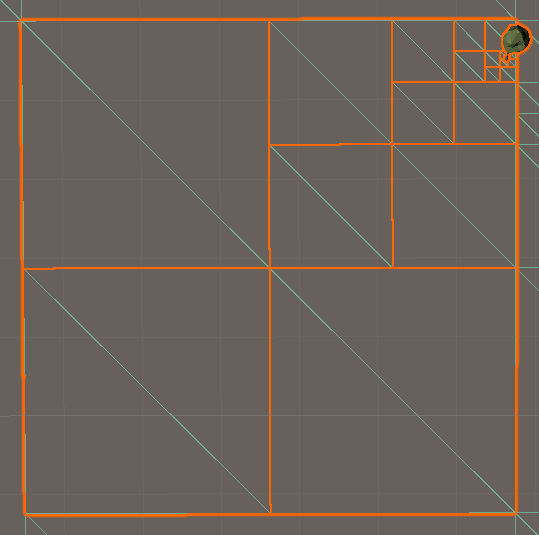Insert : This method is called for every point to check if the point lies in the specific quad or not. If the point lies in the quad or inside the bounds of the quad, then the data is added to the specific node. To further detail out, once the point is added to “Points” property, a check is performed if the number of “Points” are less than “MaxPoints”. Based on the result, the quad is further subdivided.

Quick optimization: Always check first if the point lies in the quad or not and if not, perform an early return. This will save lot of calculations.

Quad : This is the constructor of the class. As simple as it can be, just initializes the class and properties if required.

Subdivide : This is the function which is directly called if the “Points” outrun the “MaxPoints” count. This calls the “CreateQuad” function 4 times to create 4 different quads by subdividing the current quad’s area/bounds into 4 equal parts.

# What next?

Once we have the class, we need to implement and start filling up the data. As we know that we need to have a root node. The user here has a choice to implement this in various ways. User can dynamically create multiple quadtrees at runtime or mark a whole area and do the quadtree partitioning. To make this very simple, I have taken up a 3d scene and the extents of the same. Then I created a “RootNode” and provided the bounds for the same.

As you can see in the image below, I have marked the root node and given a bounds of 500x500. This is huge looking at the 3d data I have, but makes it simpler to understand in this article: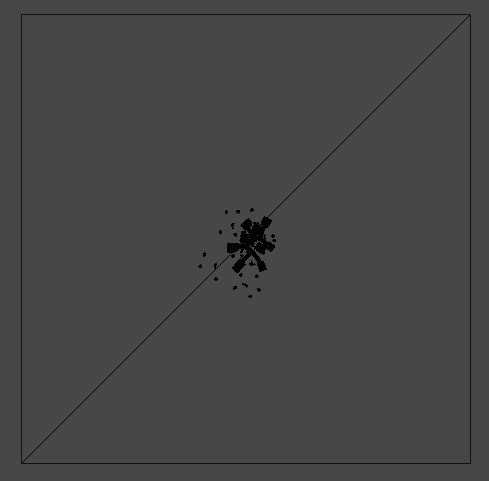# Sorting The Data

For this article, the data is the mesh. Hence, for each vertex in the scene, we will call the “Insert” method of the RootNode. If the root contains the vertex position, then it will add the point to its data, else will ignore it (here the dynamic creation of quads will be more effective).

If the number of points exceed, the root node will be subdivided into 4 parts and for-each the same “Insert” method will be called. Remember that each quad has the Insert function! The following image will help you understand the quads that were created: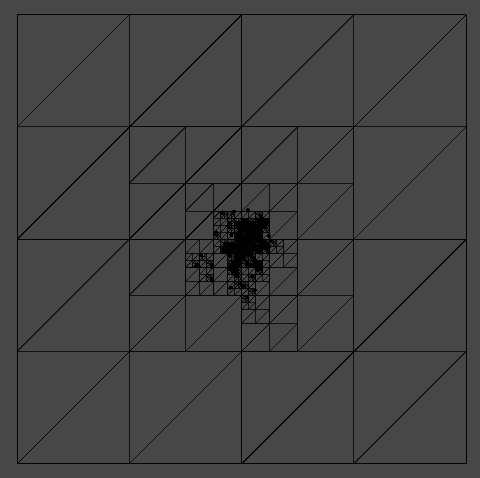I have deliberately left a lot of free space so that we can see that where there is no or less data, the bounds of the quads are larger. This proves, that if the data overflows, then and only then, the quad will be subdivided. Here, I have kept the limit of 250 vertices per quad. We can clearly see the area highlighted in blue has more vertex, hence its further subdivided, whereas the other area has larger quads: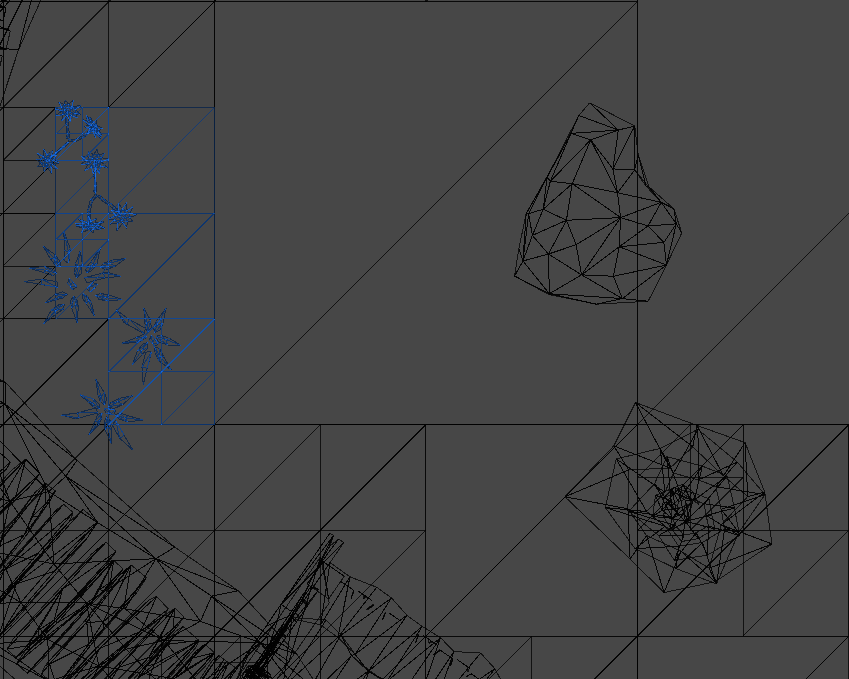Quick Tip : In unity the rectangle class takes x and y parameters only. Hence, for a 3d point, we can take only 2 axis. Here I have taken X and Z axis.

# Conclusion

So here we conclude with the creation and implementation of the QuadTree data structure. We have seen the implementation and the uses also. Apart from what I have discussed, this can be used at a lot of places. Ideally terrain generation is the best example that can be given, where based on the distance of the player, the quads are subdivided to render higher detail. Those away from the user will be showing less data.

Hope this simplified version helps you to understand and implement your own Quadtree structure.

### Latest Jobs

#### Manticore Games

San Mateo, California
8.23.22
Senior Software Engineer - Mobile

#### Sony PlayStation

San Diego, California
6.23.22
Sr. Online Programmer

#### The Walt Disney Company

Glendale, California
8.1.22
Associate Marketing Manager - Walt Disney Games

#### Insomniac Games

Burbank, California
8.26.22
Accessibility Design Researcher
More Jobs

#### Game Developer Job Board

Browse open positions across the game industry or recruit new talent for your studio

#### Game Developer Newsletter

Get daily Game Developer top stories every morning straight into your inbox